Thank you for your query. We will reply to you at the earliest.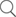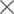## Exploring Forward Error Correction Trends in Ethernet

Resources >> Articles >> Exploring Forward Error Correction Trends in Ethernet

### Exploring Forward Error Correction Trends in Ethernet

Since the advent of electronics, the need for high-speed data communication has been growing. Particularly the rise in prominence of cloud computing, IOT and massive sized media files has simply increased the demands on the networks to support higher speeds and evolve with the demands of the current age and time.

The Ethernet protocol (IEEE Std 802.3) is a networking protocol conforming to the OSI networking model has also evolved across the ages. It has moved from supporting speeds of 10Mbps,1Gbps to currently offering high-speed connectivity as high as 100Gbps, 200Gbps and 400Gbps depending on individual network requirements. In day to day life, Ethernet is used in Local Area Networks(LAN's), Metropolitan Area Networks(MAN's) and Wide Area Networks(WAN's). It provides support for several types of medium for final bus level transmissions such as support for backplanes, co-axial cables and also the up and on the rise optical networks.

The Ethernet protocol follows the ISO/IEC Open System Interconnection (OSI) reference model to implement its Media Access Control(MAC) and Physical(PHY) sub-layers. High speed Ethernets like 10/25/40/100/ G usually have the Reconciliation Sublayer(RS) acting as the bridge between the MAC and PHY sub-layers and the PHY sub-layers (which are  Physical Coding Sub-layer(PCS), Physical Medium Attachment(PMA), Physical Medium Dependent(PMD), and a Forward Error Correcting sub-layer(FEC)). The basic description of these PHY sub-layers involves; PCS performing encoding/scrambling of the data received, PMA responsible for providing an attachment unit interface and sometimes performing multiplexing/de-multiplexing, PMD connects the PHY to the medium at bus level and finally the FEC which is responsible for error detection and correction.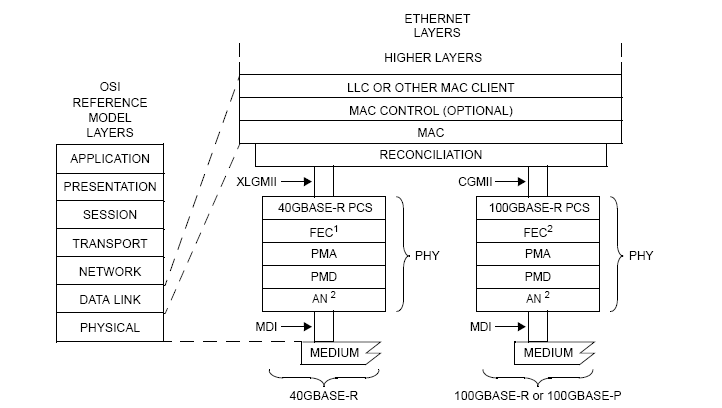Now that we have covered the basic functionality of each component, let's move over to the necessity of FEC sublayer in the PHY of any high-speed Ethernet protocol. When we work on such high speeds we have to be careful regarding the Bit Error Ratio(BER) and the integrity of the system at work. The integrity is maintained by the MAC control and other higher layers which have acknowledgment packets in place to take care of that, but to maintain a low BER we have to have a system in place which can detect and correct few error in a large chunk of data being transmitted between two DTE. The correction part is also necessary because too many re-transmissions also diminishes the purpose of having a high-speed transmitter/receiver. So it is better to have an FEC capable of correcting more number of errors.

To see how FEC's used in Ethernet can detect and correct errors, it is important to understand the type of error that is added to the bits being transmitted. The error types can be broadly classified into two kinds. Firstly the type of error that is localized to a certain region of the information being sent i.e. it is localized to a 'burst' or group of bits. The second kind is when the error is scattered throughout the codeword not confined to a certain region. The approach while going for the detection/correction of both the kinds of errors is quite different although the steps involved can broadly be seen to be the same.

Now that a basic understanding of the necessity of FEC's has been established, we need to understand how they work. So we'll go through some basics of Linear codes and Cyclic codes.

The information being transmitted between two DTE is sent in specific frame formats of data bits. In linear block coding, this is actually helpful, where this same binary information is segmented into message blocks of fixed length; which consists of k information bits. The encoder depending on some protocol and set of rules transforms this message into a binary n-tuple which referred to as code word of the message and is represented as (n,k), where n is the length of the codeword and k is the length of the message (n>k always).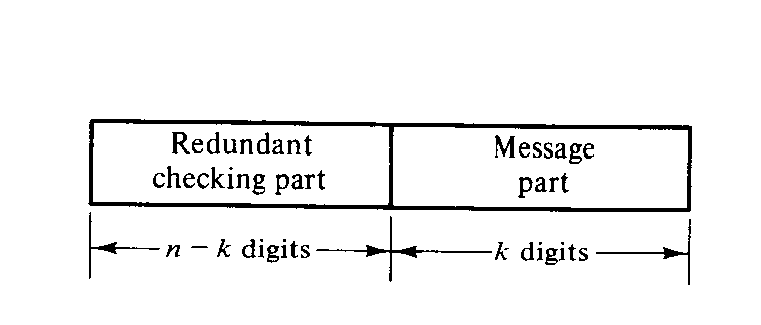As the figure shows the message part consists of k unaltered information (or message) bits and redundant checking part consists of n-k parity check bits, which are linear sums of the information bits. The codewords generated for a specific message block are distinct and have a one to one correspondence to the message block, which is an important property for error detection and correction as there are 2^k specific code words for any specific encoder which are correct and the rest are incorrect. The basic maths involved in generating linear block codes is the multiplication of the message block and the generator matrix, where the generator matrix consists of Parity matrix and k*k identity matrix.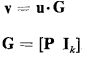Where u is the message information, G is the generator matrix, P is Parity check matrix and I the identity matrix

Now when this message is transmitted over a noisy channel there are chances of corruption in the codeword received from the other terminal. The decoder on the far-end terminal must first detect the errors and then it should either takes actions to locate the error and correct them (which is actually the FEC functionality) or request for retransmission. The detection process involves generating the syndrome of the received vector which is the remainder obtained by dividing the received vector by the generator matrix or performing the vector sum of received parity bits and parity check digits. Then syndrome is zero if and only if the received vector is a codeword, and it's not zero if and only if the received vector is not a codeword, therefore indicating the presence of an error in the received vector. Now the syndrome vector generated can have 2^k solutions. Hence the error correction method has to determine the true error vector from a set of 2^k candidates after that just add the error vector to the received vector and you get the corrected codeword. Now the process involved in determining the correct error vector is different for different encoding scheme which we shall encounter here.

Few points on the error detection and correction, it's possible that the errors in certain error vectors are not detectable, which happens when the error pattern is identical to a non-zero code word, as it's known that the sum of two codewords is a new codeword the resultant of such error is also code word which goes undetectable. Also a block code with the minimum distance between two codewords dmin guarantees correcting all the error patterns of or  fewer errors where t is called the random-error-correcting capability of the code.

Moving on to a rather interesting subclass of linear codes which are cyclic codes. They were used widely because of 2 reasons, first being the use of shift registers with feedback connections for encoding and syndrome computation purposes; and second, as they had a good algebraic structure, it was possible to find a suitable decoding method for them. This is all possible because of the basic property of cyclic code words, that is if any codeword is shifted cyclically, the result is also a codeword, which means that all codewords can be generated from a single sequence by the processes of shifting and addition.

The process of encoding and decoding for cyclic codes consists of division of the message block by generator polynomial to obtain the remainder which is appended to the message block to produce the codeword and at the receiver end division of the received vector with the generator polynomial to obtain the remainder which is, in turn, the syndrome, but it's all done by modulo 2 arithmetic which can be easily performed with the help of shift register as shown in the figures.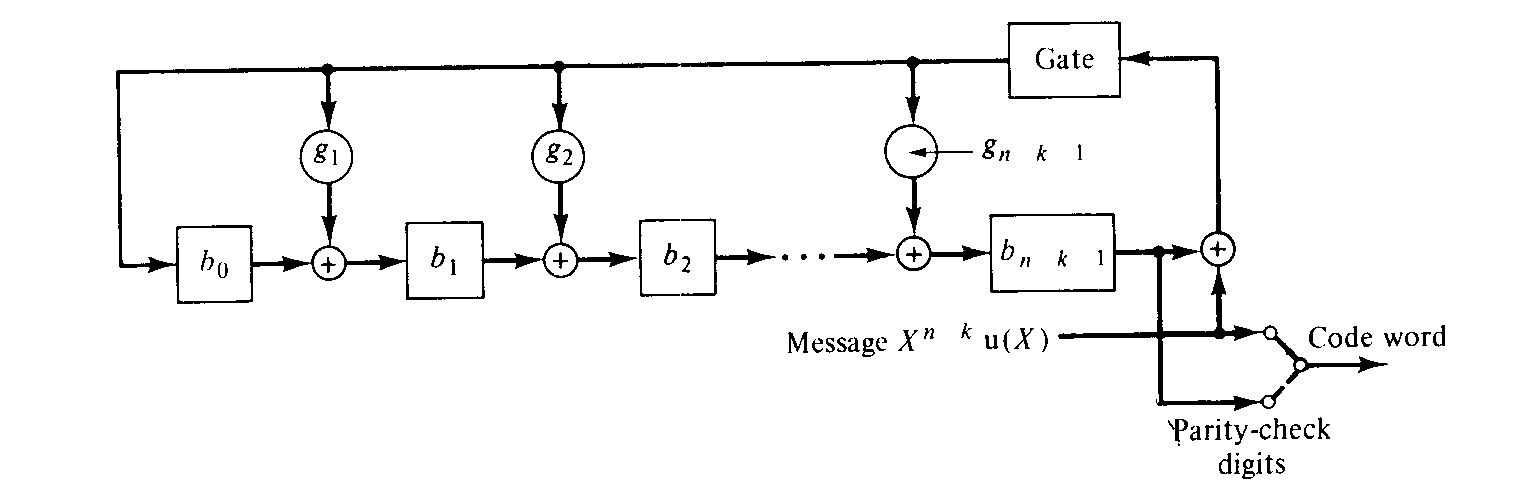Now the problem with cyclic codes is that they add too much overhead of redundant information and if there are bandwidth limitations to be met. In some systems, it might be suitable to shorten the above mentioned cyclic codes to fit the purpose of use. A shortened cyclic code implies that the previously mentioned (n,k) cyclic code has been reduced to (n-l,k-l) linear code of 2^(k-l) vectors i.e. it has been shortened by a factor of l. The shortening of the cyclic code points to the upper order bits of the information word considered being zero. The same circuitry as used with cyclic codes can be used for syndrome calculation and decoding of these shortened cyclic code words, taking into account the now extra l digits (ex: by incorporating extra shifts if implementation was done using shift registers)in the new codeword. In many cases, as this might pose an unnecessary overhead, some modifications can be made as well to the circuitry for efficient processing of the shortened cyclic codes.

An example of the implementation using the aforementioned shortened cyclic code is the FEC sub-layer for BASE-R PHY's. This FEC uses a (2112,2080) codeword that has been shortened from the (42987,42955) cyclic code and is capable of correcting a 11-bit burst error. A 11-bit burst error can be understood to be a set of consecutive bits where the number of error bits can range from one to eleven. When the entire received vector has been obtained the first and foremost step involved in the FEC on the reception side is the calculation of the syndrome. As mentioned previously a non-zero value indicates the presence of errors in the received vectors in which case the next step involved is finding the received vector bits with the error. While going for burst error detection/correction we try to confine the burst error in a fixed bit window by means of a series of shifts. Post each shift, the changing value of the syndrome is monitored. Let the syndrome value associated with the maximum error, that in this case is a 11bit burst error with an error in each bit is the threshold value 't'. The error can be assumed to have been trapped when the syndrome value being monitored is less than or equal to 't'. By the time the entire received vector has been traversed if this condition is never found to have been satisfied then further steps for negative acknowledgment or retransmission need to be taken as corrupted but uncorrected bits were received.

A major drawback of this type of FEC apart from being limited to maximum 11-bit correction, is that it is built for errors which are confined close together in this particular case a consecutive 11-bit window. This might not always be the case as more often than not the errors tend to be scattered through the received vector.

To overcome some of the limitations Ethernet faced in BASE-R FEC, the high speed Ethernet moved over to Reed Solomon coding schemes for performing Forward Error Correction as it has very high error detection, correction capabilities with a smaller overhead caused by redundant information, and as the benefits increase so does the encoding and decoding complexities which requires strong background in maths. The Reed Solomon codes work on Galois Field arithmetic. A base 2 finite field, is also known as a Galois field. A finite field contains a set of pre-determined elements. The field is constructed using prime number base, as it produces unique value when added, multiplied to any other field elements. This coding scheme follows modulo addition/multiplication. For subtraction and division, we calculate the additive inverse and multiplicative inverse to get the resultant. Galois field is denoted as GF(2^n) where n is the number of elements in the field. The primitive polynomial helps in generating the elements and performing basic arithmetic operations.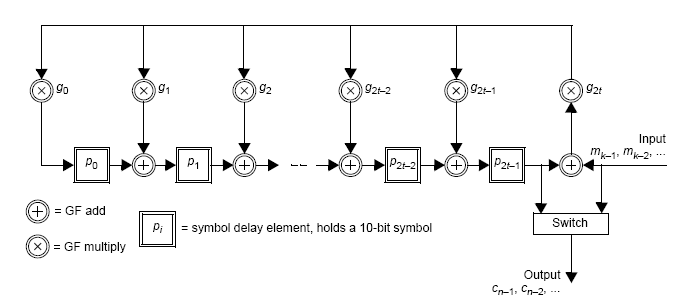The Reed Solomon encoder used in 100G Ethernet is RS(528,514) which operates over the Galois field GF(2^10) where the symbol size is 10 bits, k is 514 symbols and n is 528 symbols. The decoder is capable of correcting [(n-k)/2]=t=7 symbols in the codeword. The encoder divides the Message block by Generator Polynomial using the Galois field arithmetic which produces a parity polynomial and which in turn is appended to the message block to produce the codeword. The decoding part is where there's a lot of work involved. Although the Syndrome is the remainder of the division of the received vector by the generator polynomial, it's a tedious job to do, so we calculate the syndrome by substituting the finite field roots a(n) into the received vector R(X) to produce the syndrome coefficients. which can be further made easy by using the Horner's algorithm. After we compute the Syndrome, we have to figure out the error magnitude and the error location. The error magnitude polynomial determines how many errors are present in the received codeword. These two polynomials are computed by dividing a X^2t polynomial by the syndrome polynomial using Euclidean long division method, where the remainder is error magnitude polynomial and the quotient is the error locator polynomial. These two polynomials are further used by the Chien search algorithm to actually identify the error locations, and finally, Forney algorithm is used to determine the error vector for specific locations.

This is the most advanced encoding scheme used in Ethernet yet to perform forward error correction, in terms of correction capabilities of up to 7 symbols or 70 bits with a very small overhead. However this also has a disadvantage when there are more than 7 symbol errors spread across the codeword, the Chien search algorithm churns out invalid data locations and after this, if correction is attempted, the received vector was further seen to be corrupted. And Truechip has devised a way to circumvent this false correction when no correction is feasible.

In many FEC sub layer of the Ethernet PHY, an option can be provided to bypass the correction phase altogether as the implementer might not want their performance objectives to be hindered with. In such cases, a provision can be made to communicate the presence of the error to the next PHY sub layer in the data flow path.

As seen, the effectiveness of the FEC components is limited by several factors. In the future as the need arises the capabilities of these components can be further enhanced. Currently, the RS-FEC design proposed for the 200G and 400G Ethernet utilizes a (544,514) code increasing the number of parity symbols to 30 from 14, thus increasing the error correction capability from 7 to 15 symbols. Also, newer algorithms that rely on the efficient use of parallelism can reduce the computational load on systems and increase the speed of error detection/correction in high-speed networks.